Next: Starting and safe-guarding Newton's Up: The Trust-Region subproblem Previous: Explanation of the Hard   Contents

# Finding the root of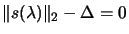.

We will apply the 1D-optimization algorithm called 1D Newton's search'' (see Annexes, Section 13.5) to the secular equation: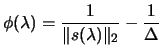(4.14)

We use the secular equation instead of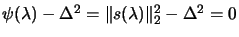inside the 1D Newton's search'' because this last function is better behaved than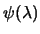. In particular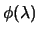is strictly increasing when, and concave. It's first derivative is: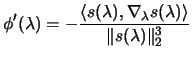(4.15)

where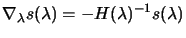(4.16)

The proof of these properties will be skipped.
In order to apply the 1D Newton's search'':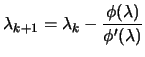(4.17)

we need to evaluate the functionand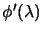. The value ofcan be obtained by solving the Equation 4.9 to obtain. The value ofis available from 4.17 oncehas been found using 4.18. Thus both values may be found by solving linear systems involving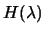. Fortunately, in the range of interest,is definite positive, and thus, we may use its Cholesky factors(see Annexes, Section 13.7 for notes about Cholesky decomposition ). Notice that we do not actually need tho find, but merely the numerator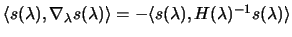of 4.17. The simple relationship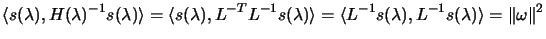(4.18)

explains why we compute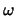in step 3 of the following algorithm. Step 4 of the algorithm follows directly from 4.17 and 4.19. Newton's method to solve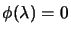:
1. find a value of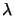such thatand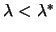.
2. factorize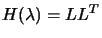3. solve4. Solve5.  Replaceby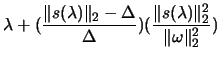(4.19)

6. If stopping criteria are not met, go to step 2.
Once the algorithm has reached point 2. It will always generate values of. Therefore, the Cholesky decomposition will never fails and the algorithm will finally find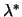. We skip the proof of this property.Next: Starting and safe-guarding Newton's Up: The Trust-Region subproblem Previous: Explanation of the Hard   Contents
Frank Vanden Berghen 2004-04-19